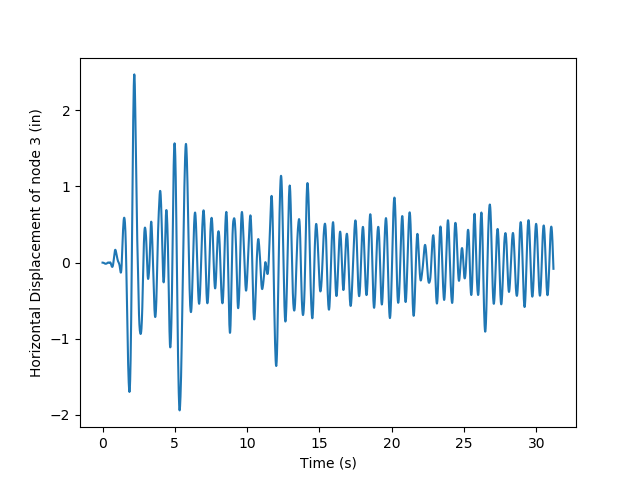# 14.2.2. Reinforced Concrete Frame Earthquake Analysis¶

1. The source code is shown below, which can be downloaded `here`.

2. The file for gravity analysis is also needed `here`.

3. The ReadRecord is a useful python function for parsing the PEER strong motion data base files and returning the `dt`, `nPts` and creating a file containing just data points. The function is kept in a seperate file `here` and is imported in the example.

4. The ground motion data file `here` must be put in the same folder.

5. Run the source code in your favorite Python program and should see `Passed!` in the results and a plotting of displacement for node 3```  1print("==========================")
2print("Start RCFrameEarthquake Example")
3
4# Units: kips, in, sec
5#
6# Written: Minjie
7
8from openseespy.opensees import *
9
11import numpy as np
12import matplotlib.pyplot as plt
13
14wipe()
15# ----------------------------------------------------
16# Start of Model Generation & Initial Gravity Analysis
17# ----------------------------------------------------
18
19# Do operations of Example3.1 by sourcing in the tcl file
20import RCFrameGravity
21print("Gravity Analysis Completed")
22
23# Set the gravity loads to be constant & reset the time in the domain
25
26# ----------------------------------------------------
27# End of Model Generation & Initial Gravity Analysis
28# ----------------------------------------------------
29
30# Define nodal mass in terms of axial load on columns
31g = 386.4
32m = RCFrameGravity.P/g
33
34mass(3, m, m, 0.0)
35mass(4, m, m, 0.0)
36
37# Set some parameters
38record = 'elCentro'
39
40# Permform the conversion from SMD record to OpenSees record
42
43# Set time series to be passed to uniform excitation
44timeSeries('Path', 2, '-filePath', record+'.dat', '-dt', dt, '-factor', g)
45
47#                         tag dir
48pattern('UniformExcitation',  2,   1,  '-accel', 2)
49
50# set the rayleigh damping factors for nodes & elements
51rayleigh(0.0, 0.0, 0.0, 0.000625)
52
53# Delete the old analysis and all it's component objects
54wipeAnalysis()
55
56# Create the system of equation, a banded general storage scheme
57system('BandGeneral')
58
59# Create the constraint handler, a plain handler as homogeneous boundary
60constraints('Plain')
61
62# Create the convergence test, the norm of the residual with a tolerance of
63# 1e-12 and a max number of iterations of 10
64test('NormDispIncr', 1.0e-12,  10 )
65
66# Create the solution algorithm, a Newton-Raphson algorithm
67algorithm('Newton')
68
69# Create the DOF numberer, the reverse Cuthill-McKee algorithm
70numberer('RCM')
71
72# Create the integration scheme, the Newmark with alpha =0.5 and beta =.25
73integrator('Newmark',  0.5,  0.25 )
74
75# Create the analysis object
76analysis('Transient')
77
78# Perform an eigenvalue analysis
79numEigen = 2
80eigenValues = eigen(numEigen)
81print("eigen values at start of transient:",eigenValues)
82
83# set some variables
84tFinal = nPts*dt
85tCurrent = getTime()
86ok = 0
87
88time = [tCurrent]
89u3 = [0.0]
90
91# Perform the transient analysis
92while ok == 0 and tCurrent < tFinal:
93
94    ok = analyze(1, .01)
95
96    # if the analysis fails try initial tangent iteration
97    if ok != 0:
98        print("regular newton failed .. lets try an initail stiffness for this step")
99        test('NormDispIncr', 1.0e-12,  100, 0)
100        algorithm('ModifiedNewton', '-initial')
101        ok =analyze( 1, .01)
102        if ok == 0:
103            print("that worked .. back to regular newton")
104        test('NormDispIncr', 1.0e-12,  10 )
105        algorithm('Newton')
106
107    tCurrent = getTime()
108
109    time.append(tCurrent)
110    u3.append(nodeDisp(3,1))
111
112
113
114# Perform an eigenvalue analysis
115eigenValues = eigen(numEigen)
116print("eigen values at end of transient:",eigenValues)
117
118results = open('results.out','a+')
119
120if ok == 0:
121    results.write('PASSED : RCFrameEarthquake.py\n');
122    print("Passed!")
123else:
124    results.write('FAILED : RCFrameEarthquake.py\n');
125    print("Failed!")
126
127results.close()
128
129plt.plot(time, u3)
130plt.ylabel('Horizontal Displacement of node 3 (in)')
131plt.xlabel('Time (s)')
132
133plt.show()
134
135
136
137print("==========================")
```